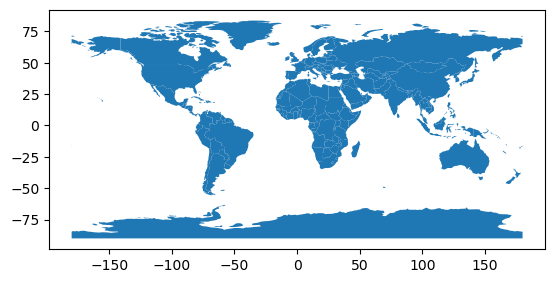Learning Objectives

• Create a Geopandas GeoSeries and Dataframe

• Plot a basic map

Review

# Spatial Vector Data#

## Intro to GeoPandas#

The goal of GeoPandas is to make working with spatial data in python easier. It combines the capabilities of pandas and shapely, providing spatial operations in pandas and a high-level interface to multiple geometries to shapely. GeoPandas enables you to easily do operations in python that would otherwise require a spatial database such as PostGIS.

## Data Structures#

GeoPandas implements two main data structures, a GeoSeries and a GeoDataFrame. These are subclasses of pandas Series and DataFrame, respectively.

### GeoSeries#

A GeoSeries is essentially a vector where each entry in the vector is a set of shapes corresponding to one observation. An entry may consist of only one shape (like a single polygon) or multiple shapes that are meant to be thought of as one observation (like the many polygons that make up the State of Hawaii or a country like Indonesia).

geopandas has three basic classes of geometric objects (which are actually shapely objects):

• Points / Multi-Points

• Lines / Multi-Lines

• Polygons / Multi-Polygons

import geopandas
from shapely.geometry import Point
s = geopandas.GeoSeries([Point(1, 1), Point(2, 2), Point(3, 3)])
s

0    POINT (1.00000 1.00000)
1    POINT (2.00000 2.00000)
2    POINT (3.00000 3.00000)
dtype: geometry

from shapely.geometry import LineString
l= geopandas.GeoSeries([LineString([Point(-77.036873,38.907192), Point(-76.612190,39.290386,), Point(-77.408456,39.412006)])])
l

0    LINESTRING (-77.03687 38.90719, -76.61219 39.2...
dtype: geometry

from shapely.geometry import Polygon
p= geopandas.GeoSeries([Polygon([Point(-77.036873,38.907192), Point(-76.612190,39.290386,), Point(-77.408456,39.412006)])])
p

0    POLYGON ((-77.03687 38.90719, -76.61219 39.290...
dtype: geometry


Note that all entries in a GeoSeries need not be of the same geometric type, although certain export operations will fail if this is not the case.

### GeoDataFrame#

A GeoDataFrame is a tabular data structure that contains a GeoSeries.

The most important property of a GeoDataFrame is that it always has one GeoSeries column that holds a special status. This GeoSeries is referred to as the GeoDataFrame’s “geometry”. When a spatial method is applied to a GeoDataFrame (or a spatial attribute like area is called), this commands will always act on the “geometry” column.

The “geometry” column – no matter its name – can be accessed through the geometry attribute (gdf.geometry), and the name of the geometry column can be found by typing gdf.geometry.name.

Note

A GeoDataFrame may also contain other columns with geometrical (shapely) objects, but only one column can be the active geometry at a time. To change which column is the active geometry column, use the GeoDataFrame.set_geometry() method.

An example using the worlds GeoDataFrame:

world = geopandas.read_file(geopandas.datasets.get_path('naturalearth_lowres'))

world.plot()

<AxesSubplot:>